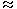# Data Interpretation - Table Charts

## Why Data Interpretation Table Charts?

In this section you can learn and practice Data Interpretation Questions based on "Table Charts" and improve your skills in order to face the interview, competitive examination and various entrance test (CAT, GATE, GRE, MAT, Bank Exam, Railway Exam etc.) with full confidence.

## Where can I get Data Interpretation Table Charts questions and answers with explanation?

IndiaBIX provides you lots of fully solved Data Interpretation (Table Charts) questions and answers with Explanation. Solved examples with detailed answer description, explanation are given and it would be easy to understand. All students, freshers can download Data Interpretation Table Charts quiz questions with answers as PDF files and eBooks.

## Where can I get Data Interpretation Table Charts Interview Questions and Answers (objective type, multiple choice)?

Here you can find objective type Data Interpretation Table Charts questions and answers for interview and entrance examination. Multiple choice and true or false type questions are also provided.

## How to solve Data Interpretation Table Charts problems?

You can easily solve all kind of Data Interpretation questions based on Table Charts by practicing the objective type exercises given below, also get shortcut methods to solve Data Interpretation Table Charts problems.

### Exercise :: Table Charts - Table Chart 1

Study the following table and answer the questions based on it.

Expenditures of a Company (in Lakh Rupees) per Annum Over the given Years.

 Year Item of Expenditure Salary Fuel and Transport Bonus Interest on Loans Taxes 1998 288 98 3.00 23.4 83 1999 342 112 2.52 32.5 108 2000 324 101 3.84 41.6 74 2001 336 133 3.68 36.4 88 2002 420 142 3.96 49.4 98

1.

What is the average amount of interest per year which the company had to pay during this period?

 A. Rs. 32.43 lakhs B. Rs. 33.72 lakhs C. Rs. 34.18 lakhs D. Rs. 36.66 lakhs

Explanation:

Average amount of interest paid by the Company during the given period

 = Rs.23.4 + 32.5 + 41.6 + 36.4 + 49.4lakhs 5

 = Rs.183.3lakhs 5

= Rs. 36.66 lakhs.

2.

The total amount of bonus paid by the company during the given period is approximately what percent of the total amount of salary paid during this period?

 A. 0.1% B. 0.5% C. 1% D. 1.25%

Explanation:

Required percentage
 =(3.00 + 2.52 + 3.84 + 3.68 + 3.96) x 100% (288 + 342 + 324 + 336 + 420)
 =17 x 100% 17101%.

3.

Total expenditure on all these items in 1998 was approximately what percent of the total expenditure in 2002?

 A. 62% B. 66% C. 69% D. 71%

Explanation:

Required percentage
 =(288 + 98 + 3.00 + 23.4 + 83) x 100% (420 + 142 + 3.96 + 49.4 + 98)
 =495.4 x 100% 713.3669.45%.

4.

The total expenditure of the company over these items during the year 2000 is?

 A. Rs. 544.44 lakhs B. Rs. 501.11 lakhs C. Rs. 446.46 lakhs D. Rs. 478.87 lakhs

Explanation:

Total expenditure of the Company during 2000

= Rs. (324 + 101 + 3.84 + 41.6 + 74) lakhs

= Rs. 544.44 lakhs.

5.

The ratio between the total expenditure on Taxes for all the years and the total expenditure on Fuel and Transport for all the years respectively is approximately?

 A. 4:7 B. 10:13 C. 15:18 D. 5:8

Explanation:

Required ratio
 =(83 + 108 + 74 + 88 + 98)(98 + 112 + 101 + 133 + 142)
 =451586
 = 1 1.3
 = 10 . 13